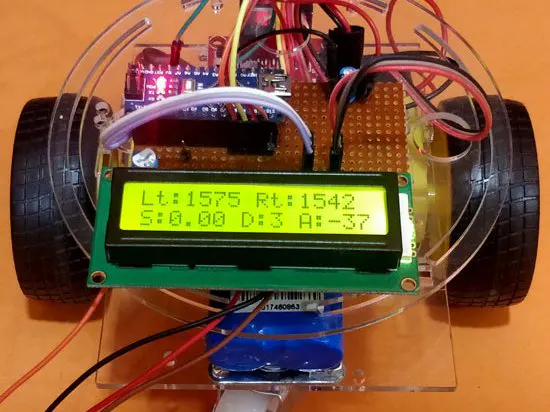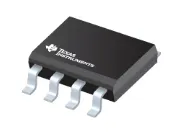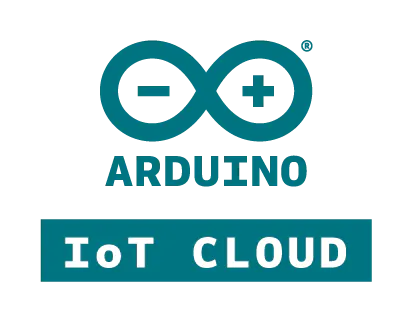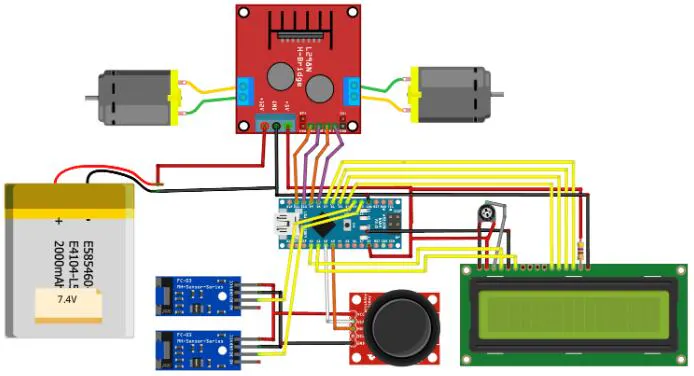# Measurement of Mobile Robots with Arduino and LM393 Sensors

This project can help you measure the speed, distance and angle of the robot.## Things used in this project

### Hardware componentsTexas Instruments LM393 chip
×1

### Software apps and online servicesArduino IoT Cloud

### Hand tools and fabrication machinesSolder Pot, LCD Display

## Schematics

### schematic_pEct78xgP9.jpg## Code

### Untitled file

Arduino
```#define LM_pos 9       // left motor
#define LM_neg 8       // left motor
#define RM_pos 10       // right motor
#define RM_neg 11       // right motor
#define joyX A2
#define joyY A3
void setup()
{
rotation = rpm = pevtime = 0; //Initialize all variable to zero
Serial.begin(9600);
lcd.begin(16, 2); //Initialise 16*2 LCD
lcd.print("Bot Monitor"); //Intro Message line 1
lcd.setCursor(0, 1);
lcd.print("-CircuitDigest "); //Intro Message line 2
delay(2000);
lcd.clear();
lcd.print("Lt:     Rt:    ");
lcd.setCursor(0, 1);
lcd.print("S:     D:  A:   ");

pinMode(LM_pos, OUTPUT);
pinMode(LM_neg, OUTPUT);

pinMode(RM_pos, OUTPUT);
pinMode(RM_neg, OUTPUT);

digitalWrite(LM_neg, LOW);
digitalWrite(RM_neg, LOW);

attachInterrupt(digitalPinToInterrupt(2), Left_ISR, CHANGE); //Left_ISR is called when left wheel sensor is triggered
attachInterrupt(digitalPinToInterrupt(3), Right_ISR, CHANGE);//Right_ISR is called when right wheel sensor is triggered
}
void Left_ISR()
{
left_intr++;delay(10);
}

void Right_ISR()
{
right_intr++; delay(10);

rotation++;
dtime=millis();
if(rotation>=40)
{
timetaken = millis()-pevtime; //timetaken in millisec
rpm=(1000/timetaken)*60;    //formulae to calculate rpm
pevtime = millis();
rotation=0;
}
}

int acceleration = map (xValue, 500, 0, 0, 200);

if (xValue<500)
{
analogWrite(LM_pos, acceleration);
analogWrite(RM_pos, acceleration);
}

else
{
analogWrite(LM_pos, 0);
analogWrite(RM_pos, 0);
}

if (yValue>550)
analogWrite(RM_pos, 80);

if (yValue<500)
analogWrite(LM_pos, 100);
v = radius_of_wheel * rpm * 0.104; //0.033 is the radius of the wheel in meter
int angle_left = (left_intr % 360) * (90/80) ;
int angle_right = (right_intr % 360) * (90/80) ;
angle = angle_right - angle_left;

lcd.setCursor(3, 0); lcd.print("    "); lcd.setCursor(3, 0); lcd.print(left_intr);
lcd.setCursor(11, 0); lcd.print("    "); lcd.setCursor(11, 0);lcd.print(right_intr);
lcd.setCursor(2, 1); lcd.print("  "); lcd.setCursor(2, 1);lcd.print(v);
lcd.setCursor(9, 1); lcd.print("  "); lcd.setCursor(9, 1);lcd.print(distance);
lcd.setCursor(13, 1); lcd.print("   "); lcd.setCursor(13, 1);lcd.print(angle);
```